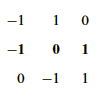# Singular Value Decomposition

« Previous | Next »

## Session OverviewIf A is symmetric and positive definite, there is an orthogonal matrix Q for which A = Q_Λ_QT. Here Λ is the matrix of eigenvalues. Singular Value Decomposition lets us write any matrix A as a product U_Σ_VT where U and V are orthogonal and Σ is a diagonal matrix whose non-zero entries are square roots of the eigenvalues of ATA. The columns of U and V give bases for the four fundamental subspaces.

## Session Activities

### Lecture Video and Summary

• Read Section 6.7 in the 4th edition or Section 7.1 and 7.2 in the 5th edition.

## Check Yourself

### Problems and Solutions

Work the problems on your own and check your answers when you’re done.

« Previous | Next »

#### Learning Resource Types

theaters Lecture Videos
grading Exams with Solutions
notes Lecture Notes
theaters Recitation Videos
assignment_turned_in Problem Sets with Solutions
laptop_windows Simulations
groups Course Introduction
co_present Instructor Insights